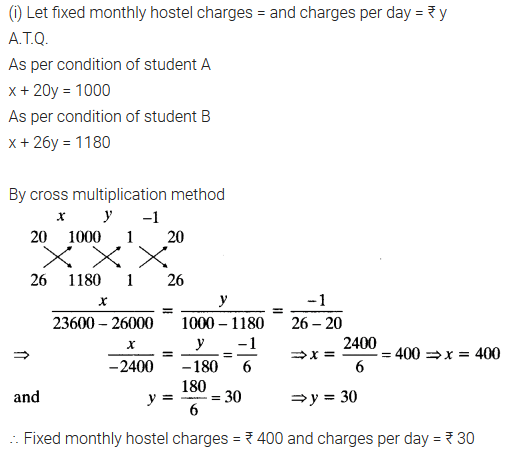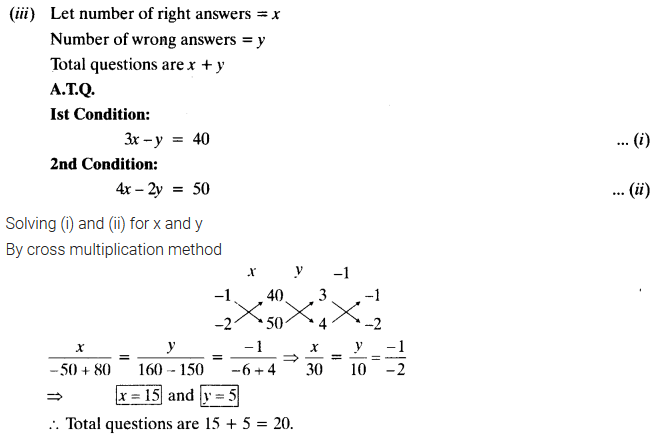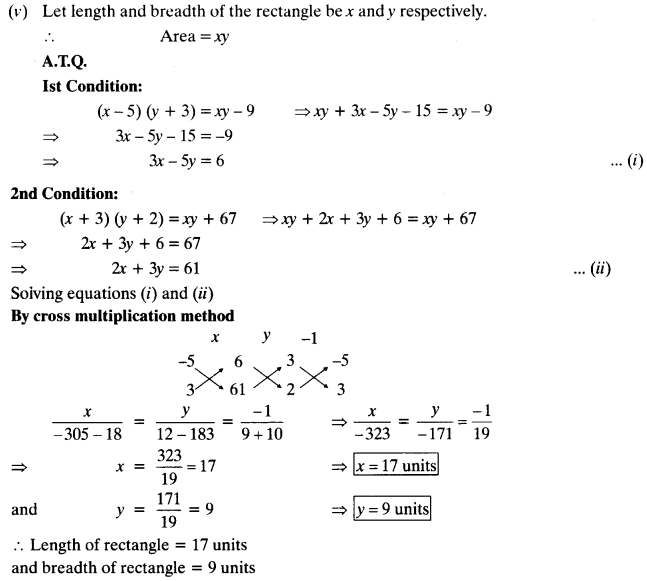ncert textbook

# NCERT Solutions for Class 10 Maths Chapter 3 Pair of Linear Equations in Two Variables Ex 3.5

Get Free NCERT Solutions for Class 10 Maths Chapter 3 Ex 3.5 PDF. Pair of Linear Equations in Two Variables Class 10 Maths NCERT Solutions are extremely helpful while doing your homework. Exercise 3.5 Class 10 Maths NCERT Solutions were prepared by Experienced ncert-books.inTeachers. Detailed answers of all the questions in Chapter 3 Maths Class 10 Pair of Linear Equations in Two Variables Exercise 3.5 provided in NCERT TextBook.

Topics and Sub Topics in Class 10 Maths Chapter 3 Pair of Linear Equations in Two Variables:

 Section Name Topic Name 3 Pair of Linear Equations in Two Variables 3.1 Introduction 3.2 Pair Of Linear Equations In Two Variables 3.3 Graphical Method Of Solution Of A Pair Of Linear Equations 3.4 Algebraic Methods Of Solving A Pair Of Linear Equations 3.4.1 Substitution Method 3.4.2 Elimination Method 3.4.3 Cross-Multiplication Method 3.5 Equations Reducible To A Pair Of Linear Equations In Two Variables 3.6 Summary

You can also download the free PDF of  Ex 3.5 Class 10 Pair of Linear Equations in Two Variables NCERT Solutions or save the solution images and take the print out to keep it handy for your exam preparation.

 Board CBSE Textbook NCERT Class Class 10 Subject Maths Chapter Chapter 3 Chapter Name Pair of Linear Equations in Two Variables Exercise Ex 3.5 Number of Questions Solved 4 Category NCERT Solutions

## NCERT Solutions for Class 10 Maths Chapter 3 Pair of Linear Equations in Two Variables Ex 3.5

NCERT Solutions for Class 10 Maths Chapter 3 Pair of Linear Equations in Two Variables Ex 3.5 are part of NCERT Solutions for Class 10 Maths. Here we have given NCERT Solutions for Class 10 Maths Chapter 3 Pair of Linear Equations in Two Variables Exercise 3.5

Question 1.
Which of the following pairs of linear equations has unique solution, no solution or infinitely many solutions. In case there is unique solution, find it by using cross-multiplication method.
(i) x – 3y – 3 = 0, 3x – 9y – 2 = 0
(ii) 2x + y = 5, 3x + 2y = 8
(iii) 3x – 5y = 20, 6x – 10y = 40
(iv) x – 3y – 7 = 0, 3x – 3y – 15 = 0
Solution: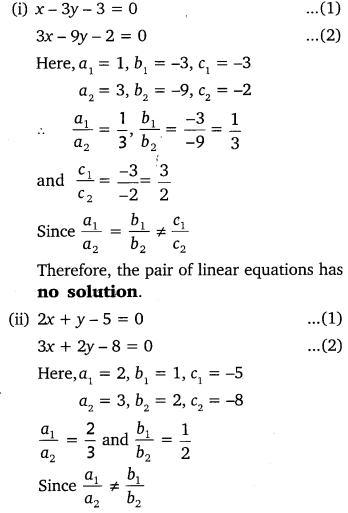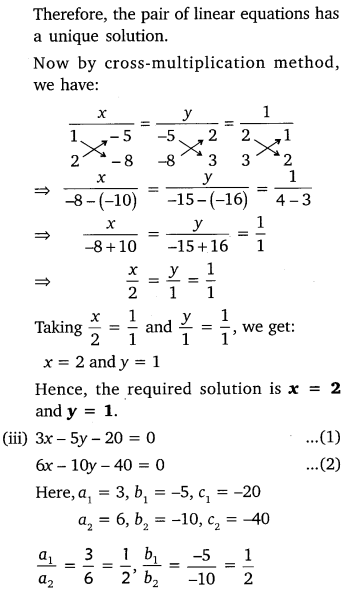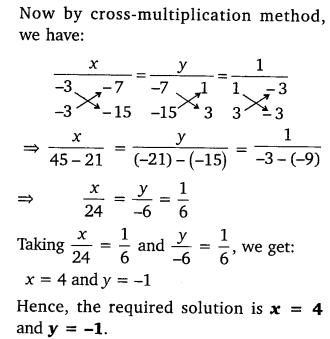Question 2.
(i) For which values of a and b does the following pair of linear equations have an infinite number of solutions?
2x + 3y = 7
(a – b)x + (a + b)y = 3a + b – 2
(ii) For which value of k will the following pair of linear equations have no solution?
3x + y = 1
(2k – 1)x + (k – 1)y = (2k + 1)
Solution:Question 3.
Solve the following pair of linear equations by the substitution and cross-multiplication methods:
8x + 5y = 9, 3x + 2y = 4
Solution: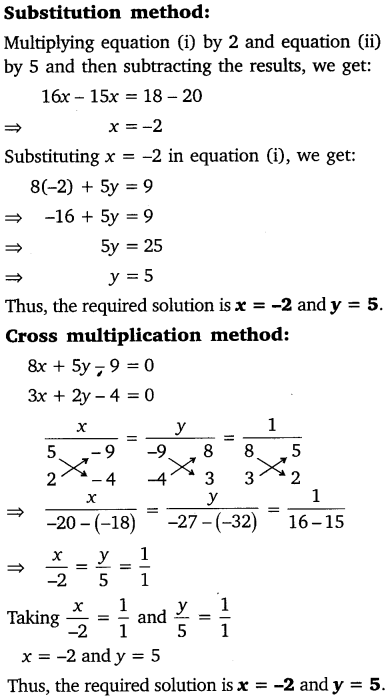Question 4.
Form the pair of linear equations in the following problems and find their solutions (if they exist) by any algebraic method:
(i) A part of monthly hostel charges is fixed and the remaining depends on the number of days one has taken food in the mess. When a student A takes food for 20 days she has to pay ₹ 1000 as hostel charges, whereas a student B, who takes food for 26 days, pays ₹ 1180 as hostel charges. Find the fixed charges and the cost of food per day.
(ii) A fraction becomes$\frac { 1 }{ 3 }$when 1 is subtracted from the numerator and it becomes$\frac { 1 }{ 4 }$when 8 is added to its denominator. Find the fraction.
(iii) Yash scored 40 marks in a test, getting 3 marks for each right answer and losing 1 mark for each wrong answer. Had 4 marks been awarded for each correct answer and 2 marks been deducted for each incorrect answer, then Yash would have scored 50 marks. How many questions were there in the test?
(iv) Places A and B are 100 km apart on a highway. One car starts from A and another from B at the same time. If the cars travel in the same direction at different speeds, they meet in 5 hours. If they travel towards each other, they meet in 1 hour. What are the speeds of the two cars?
(v) The area of a rectangle gets reduced by 9 square units, if its length is reduced by units and breadth is increased by 3 units. If we increase the length by 3 units and the breadth by 2 units, the area increases by 67 square units. Find the dimensions of the rectangle.
Solution: